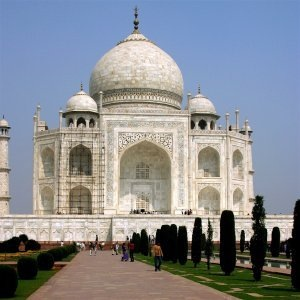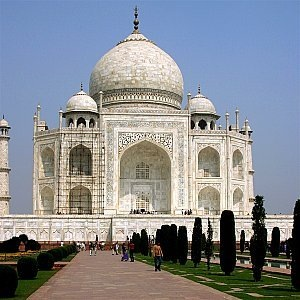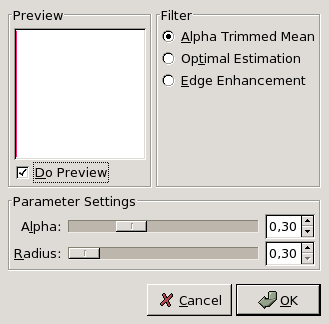On-line GuidesAll GuideseBook StoreiOS / AndroidLinux for BeginnersOffice ProductivityLinux InstallationLinux SecurityLinux UtilitiesLinux VirtualizationLinux KernelSystem/Network AdminProgrammingScripting LanguagesDevelopment ToolsWeb DevelopmentGUI Toolkits/DesktopDatabasesMail SystemsopenSolarisEclipse DocumentationTechotopia.comVirtuatopia.comHow To GuidesVirtualizationGeneral System AdminLinux SecurityLinux FilesystemsWeb ServersGraphics & DesktopPC HardwareWindowsProblem SolutionsPrivacy Policy### 3.4. NL Filter

#### 3.4.1. Overview

Figure 16.23. Example for the NL-FilterOriginal imageNL Filter” applied

NL means "Non Linear". Derived from the Unix pnmnlfilt program, it joins smoothing, despeckle and sharpen enhancement functions. It works on the whole layer, not on the selection.

This is something of a swiss army knife filter. It has 3 distinct operating modes. In all of the modes each pixel in the image is examined and processed according to it and its surrounding pixels values. Rather than using 9 pixels in a 3x3 block, it uses an hexagonal block whose size can be set with the Radius option.

#### 3.4.2. Activate the filter

You can find this filter through FiltersEnhanceNL Filter.

The filter does not work if the active layer has an alpha channel. Then the menu entry is insensitive and grayed out.

#### 3.4.3. Options

Figure 16.24. “NL Filter” optionsPreview

When checked, parameter setting results are interactively displayed in preview.

Filter

The Operating Mode described below.

Alpha

Controls the amount of the filter to apply. Valid range is 0.00-1.00. The exact meaning of this value depends on the selected operating mode. Note that this parameter is related to but not the same as the `alpha` parameter used in the pnmnlfilt program.

Controls the size of the effective sampling region around each pixel. The range of this value is 0.33-1.00, where 0.33 means just the pixel itself (and thus the filter will have no effect), and 1.00 means all pixels in the 3x3 grid are sampled.

#### 3.4.4. Operating Modes

This filter can perform several distinct functions:

Alpha trimmed mean

The value of the center pixel will be replaced by the mean of the 7 hexagon values, but the 7 values are sorted by size and the top and bottom `Alpha` portion of the 7 are excluded from the mean. This implies that an `Alpha` value of 0.0 gives the same sort of output as a normal convolution (i.e. averaging or smoothing filter), where `Radius` will determine the "strength" of the filter. A good value to start from for subtle filtering is `Alpha` = 0.0, `Radius` = 0.55. For a more blatant effect, try `Alpha` = 0.0 and `Radius` = 1.0.

An `Alpha` value of 1.0 will cause the median value of the 7 hexagons to be used to replace the center pixel value. This sort of filter is good for eliminating "pop" or single pixel noise from an image without spreading the noise out or smudging features on the image. Judicious use of the `Radius` parameter will fine tune the filtering.

Intermediate values of `Alpha` give effects somewhere between smoothing and "pop" noise reduction. For subtle filtering try starting with values of `Alpha` = 0.8, `Radius` = 0.6. For a more blatant effect try `Alpha` = 1.0, `Radius` = 1.0 .

Optimal estimation

This type of filter applies a smoothing filter adaptively over the image. For each pixel the variance of the surrounding hexagon values is calculated, and the amount of smoothing is made inversely proportional to it. The idea is that if the variance is small then it is due to noise in the image, while if the variance is large, it is because of "wanted" image features. As usual the `Radius` parameter controls the effective radius, but it probably advisable to leave the radius between 0.8 and 1.0 for the variance calculation to be meaningful. The `Alpha` parameter sets the noise threshold, over which less smoothing will be done. This means that small values of `Alpha` will give the most subtle filtering effect, while large values will tend to smooth all parts of the image. You could start with values like `Alpha` = 0.2, `Radius` = 1.0, and try increasing or decreasing the `Alpha` parameter to get the desired effect. This type of filter is best for filtering out dithering noise in both bitmap and color images.

Edge enhancement

This is the opposite type of filter to the smoothing filter. It enhances edges. The `Alpha` parameter controls the amount of edge enhancement, from subtle (0.1) to blatant (0.9). The `Radius` parameter controls the effective radius as usual, but useful values are between 0.5 and 0.9. Try starting with values of `Alpha` = 0.3, `Radius` = 0.8.

##### Combination use

The various operating modes can be used one after the other to get the desired result. For instance to turn a monochrome dithered image into grayscale image you could try one or two passes of the smoothing filter, followed by a pass of the optimal estimation filter, then some subtle edge enhancement. Note that using edge enhancement is only likely to be useful after one of the non-linear filters (alpha trimmed mean or optimal estimation filter), as edge enhancement is the direct opposite of smoothing.

For reducing color quantization noise in images (i.e. turning .gif files back into 24 bit files) you could try a pass of the optimal estimation filter (`Alpha` = 0.2, `Radius` = 1.0), a pass of the median filter (`Alpha` = 1.0, `Radius` = 0.55), and possibly a pass of the edge enhancement filter. Several passes of the optimal estimation filter with declining `Alpha` values are more effective than a single pass with a large `Alpha` value. As usual, there is a trade-off between filtering effectiveness and losing detail. Experimentation is encouraged.Published under the terms of the GNU General Public License Design by Interspire